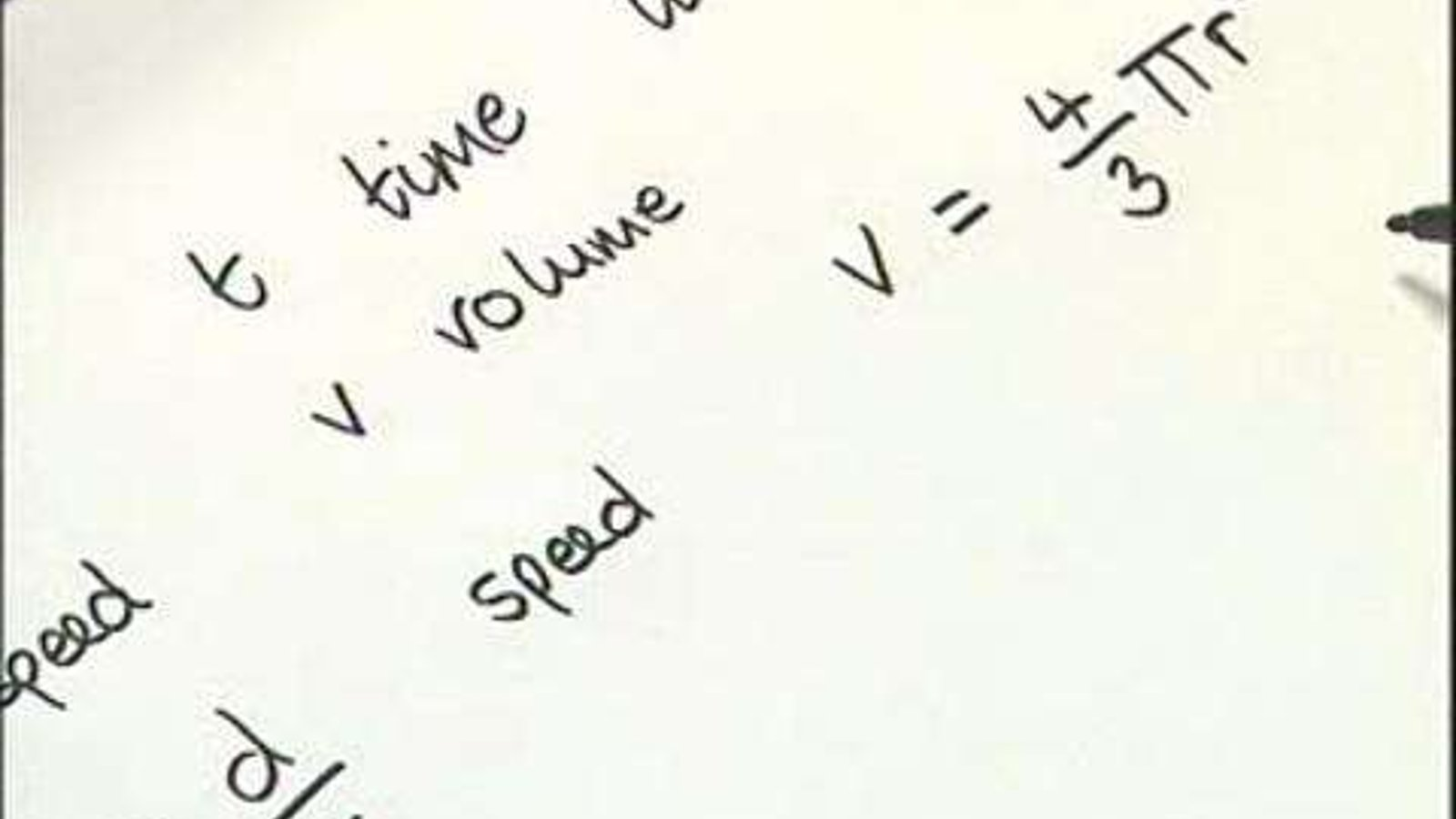# Algebra

2007The 18 videos in this collection focus on all aspects of Algebra, from logarithms to quadratic equations and partial fractions.

Supplier
FENC

### In This Series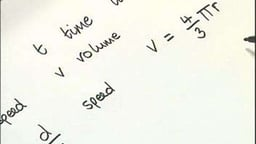Mathematical Language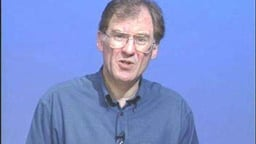Powers or Indices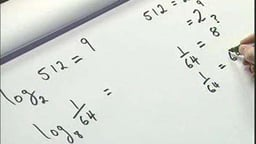Logarithms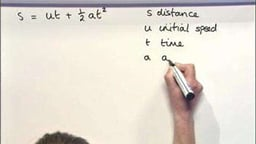Substitution and Formulae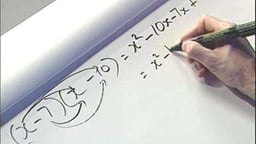Expanding and Removing Brackets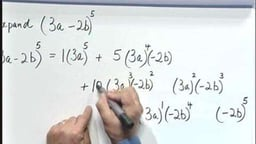Pascal's Triangle and the Binomial Theorem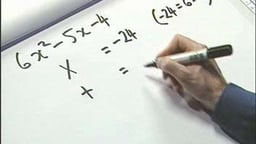Factorising Quadratics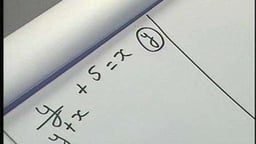Transposition of Formulae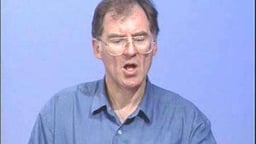Linear Equations in One Variable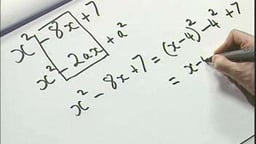Completing the SquareCompleting the Square (max, min)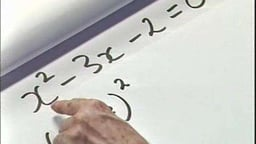Quadratic Equations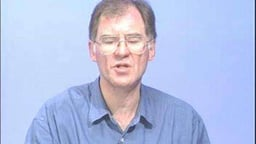Simultaneous Linear Equations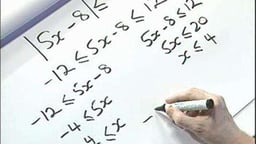Solving Inequalities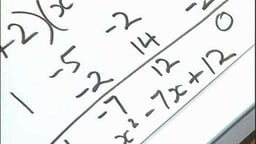Cubic EquationsSimplifying Fractions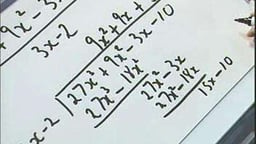Polynomial Division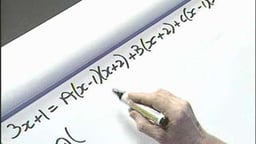Partial Fractions